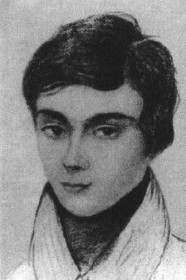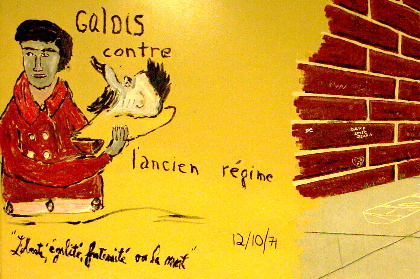# Mathematics 114 Spring, 2004## Professor Kenneth A. Ribet

email: ribet@math.berkeley.edu
Telephone: 510 642 0648
Fax: 510 642 8204
CourseWeb Math 114 Web Page
Enrollment Information

## Lectures

9 Evans Hall, TuTh 3:40-5PM. On Thursdays, come first to 1015 Evans at 3PM to have donuts and coffee.

## Syllabus

The boilerplate course description for Math 114 is as follows:
Further topics on groups, rings, and fields not covered in Math 113. Possible topics include the Sylow Theorems and their applications to group theory; classical groups; abelian groups and modules over a principal ideal domain; algebraic field extensions; splitting fields and Galois theory; construction and classification of finite fields.
The main topic of the course is undoubtedly Galois theory. We will begin by reviewing some material from Math 113 that is relevant to Galois theory and then spend the bulk of the semester on Galois theory proper. If we have time left over, we will discuss the Sylow theorems and other topics on the list that's above.

## Textbook

Before choosing this book, I considered also "Galois Theory" by Jean-Pierre Escofier, from the Springer GTM series. The Springer UTX series has a book with the same title by Joseph Rotman. These texts might be good alternative sources for some of the material that we'll be covering. The Dover book "Abstract Algebra and Solution by Radicals" by John E. Maxfield and Margaret W. Maxfield was recommended to me by Reed Harney; another promising book by the same publisher is "Galois Theory" by Emil Artin. (Please let me know if you recommend other texts on this subject that should be added to this list.)

As preparation for this class, you might enjoy reading The Galois biography on the MacTutor History of Mathematics Archive, The Galois Story by Ivars Peterson and then Tony Rothman's Genius and Biographers: The Fictionalization of Evariste Galois. (Many thanks to Moon for this suggestion.) Rothman is also the author of an earlier article about Galois (with the same title) in the American Mathematical Monthly. (To download this latter article, you need to be recognized as coming from berkeley.edu. If you're working from outside the campus network, you can use the library proxy server as long as you have a UCB library card.)

Letter grades will be a function of each student's composite numerical grade. This grade is a linear combination of the four course components: the three exams plus the aggregate homework score. The intention will be to make the course components count as follows: homework 25%, midterms 15% each, final 45%.

This class had 28 registered students (including three concurrent enrollment students). I assigned grades as follows: 17 A's (including some A+ and A- grades), 7 B's, 4 C's. One student took the course "P/NP"; the grade for that student was converted into a "P". See the grading table for more information.

## Examinations

We will have three examinations in this course:

I taught this course exactly once before: in 1992. The first midterm, second midterm and final exam from my earlier course will give you some idea of the course's content and might be of some help in preparing for the examinations in this course. The midterms from 1992 were 50 minutes along, whereas our midterms will be 80 minutes along.

## Homework

The question arises as to whether or not students are allowed to work together on problem sets. In my view, this is not only fine, but even desirable. On the other hand, writing up solutions should be a solitary activity. Discuss problems with other people until you really understand what's going on. Then you can sit down alone and write down complete solutions.

1. Assignment due January 27: Chapter 1, problems 2, 4, 7, 11. (These are on pages 14 and 15 of the book.) Before working out the problems, look over the comments page to check for misprints in the book that might affect the homework problems. In problem 4, for example, -18 should be +18 (twice).

In connection with this chapter, you might want to peek at Curt McMullen's classic article on solving the quintic equation by iteration and possibly also at Barry Mazur's recent book "Imagining Numbers (particularly the square root of minus fifteen)". An interesting review of the book by Keith Devlin is available online.

I wrote up some proposed solutions to the first homework assignment. In the future, I may be able to write up solutions to popular and/or interesting problems on assignments. If you'd like to see something solved, please let me know. As you'll see, I wrote up everything in sentences---there are formulas, but most of what you see reads like prose. This is the style that I hope you'll shoot for as well.

2. Assignment due February 3 (discussion of the extra problems about F, P, D):
• Let P(n) be as in Problem 1.11. Let F(n) be the nth Fibonacci number: F(1)=F(2)=1, F(3)=2, F(4)=3, F(5)=5, and so on. (See The First 100 Fibonacci Numbers for more values.) Consider the differences D(n) := 2P(n)-F(n+2). Tabulate enough differences D(n) so that you get a sense of the sequence {D(n)}. Prove that D(n) = D(n+1)+D(n-1) for each positive integer n and that D(n) takes only the three values 0, -1, 1.
• (continuation) Show that P(n+1)/P(n) and F(n+1)/F(n) have the same limit as n->infinity. (It is well known, and presumably proved in Math 55, that F(n+1)/F(n) approaches the Golden Mean 1.68... as n approaches infinity.)
• Problems from Chapter 2 (pp. 27-29): 2.7, 2.8, 2.14 (a-f).
• Problems from Chapter 3 (pp. 46-48): 3.1 (ace), 3.2 (ace), 3.3 (e), 3.4.
In my lecture on January 29, I mentioned "The Fundamental Theorem of Algebra" by Fine and Rosenberger. You can get some sense of it from the publisher's web page for the book.
3. Assignment due February 10 (discussion of some problems): Problems 3.5, 3.6, 3.8 (b,d,f), 3.12, 3.15, 3.16, 4.4, 4.6, 4.7.
4. Assignment due February 17: 4.8, 4.9, 4.10, 5.1, 5.2, 5.3, 5.4, 5.8, 5.9, 6.1, 6.3.
5. Assignment due February 24 (discussion of problem 6.11): 6.7, 6.8 (for polynomials of degree > 1), 6.9, 6.11, 6.12, 6.14.
6. Assignment due March 2 (discussion of some problems): 6.15, 6.16, 6.17, 8.1, 8.2, 8.5, 8.12 (parts a through d). We are going to skip chapter 7 (at least for now), discuss the first part of chapter 8 and then move on to chapter 9.
7. Assignment due March 9: 9.1, 9.2, 9.5, 9.6, 9.8, 9.9. [The grader is looking for feedback from you on the grading that she has done so far. Are her comments helpful? Should she criticize your style as well as the content? If you have feedback, please add it to the comments page.]
8. Assignment due March 16: 10.3, 10.5, 11.1, 11.2, 11.3, 11.6, 11.7.
9. Assignment due March 30 (discussion of the "part c" problems): 12.3, 12.4, 12.6, 13.1c, 13.2c, 13.3c, 13.9, 13.10.
10. Assignment due April 6: 14.1, 14.2, 14.3, 14.6, 14.7, 14.8, 14.10, 14.13.
11. Assignment due April 13: 15.1, 15.3, 15.5
12. Assignment due April 20: Let K be the extension of Q gotten by adjoining the positive square root of the number a = (6 + 2 sqrt(5)). Calculate the Galois group of the extension L:Q, where L is the splitting field of the minimal polynomial of the square root of a. List the subgroups of this Galois group and find the corresponding fixed fields. Also, do problems 15.6, 15.7, 15.9, 15.12, and problems 16.4 and 16.11 (discussion of two questions).
13. Assignment due April 27 [average 15/20]: 17.5, 17.6, 17.8, 17.9, 17.10, 17.13, 17.14.
14. Assignment due May 4: 20.1 (except for the last number); 20.2; 20.5; 20.7 for n = 8, 9, 41 and 49; 20.11; 20.12.
15. Assignment due May 11.

## Anonymous Feedback

Please let me know what I'm doing right and what I'm doing wrong. Constructive feedback is always welcome; don't hesitate to propose changes. You might be inspired by the comments that were made by students in Math H113 one year ago and by those that were made by the Math H110 students last semester.
You can read the comments that have been submitted for this course so far.